# 携程如何基于ARIMA时序分析做业务量的预测

2020 年 10 月 04 日## 一、 前言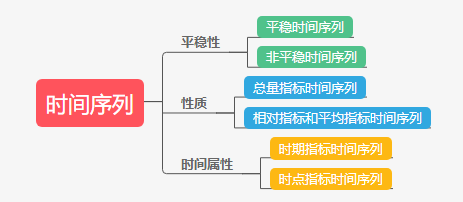• 根据指标的平稳性，分为平稳时间序列和非平稳时间序列；
• 根据指标的性质分类，分为总量指标时间序列，相对指标和平均指标时间序列；
• 根据指标的时间属性分类，分为时期指标时间序列，时点指标时间序列；

• 具有业务特征的周期性影响
• 节假日等特定时序节点的变异
• 地域差异，空间的相互作用
• 受到库存、实际市场容量的影响
• 其他外生变量，不可控自然或社会因素

## 二、 时间序列分析实践

### 2.1 ARIMA 模型简介

ARMA 模型的全称是自回归移动平均模型，可以说是目前最常用的拟合平稳序列的模型。

ARMA 模型由两部分组成：

p 阶自回归模型 AR§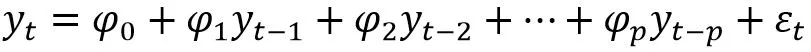φ0=0$\varphi_0=0$ 时，自回归模型又称为中心化 AR§模型。非中心化的 AR§序列也可以转化（通过平移）为中心化的 AR§模型。

AR 模型将某时刻 t 的值用过去若干时刻 t-1 到 t-p 的值通过线性组合以及噪声来表示。

q 阶移动平均模型 MA(q)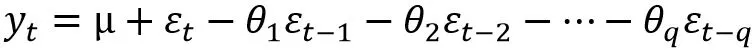μ=0$\mu=0$ 时，模型 MA(q) 称为中心化 MA(q) 模型，对于非中心化的 MA(q) 模型只要做简单的位移就可以转化为中心化的 MA(q) 模型。

MA 模型是通过历史点的噪声线性组合来表示当前时刻的值。

ARMA 模型其实就是 AR§和 MA(q) 的组合：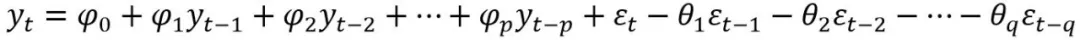### 2.2 ARIMA 模型实践分析步骤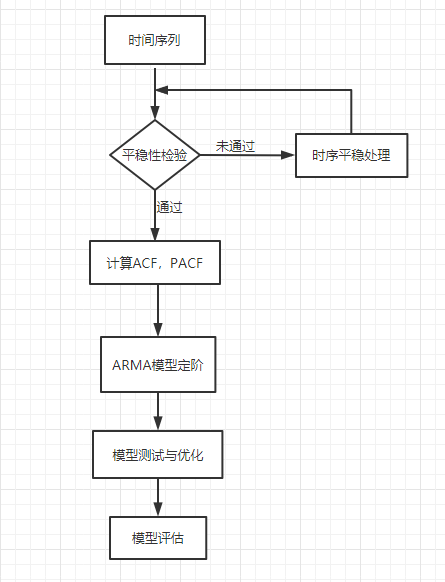Step1、读取时间序列

df = pd.read_csv('testdata.csv', encoding='gbk', index_col='ddate')#时间序列索引转换为日期格式df.index = pd.to_datetime(df.index)#指标量转为 float 类型df['cnt'] = df['cnt'].astype(float) plt.figure(facecolor='white',figsize=(20,8))plt.plot(df.index,df['cnt'],label='Time Series')plt.legend(loc='best')plt.show()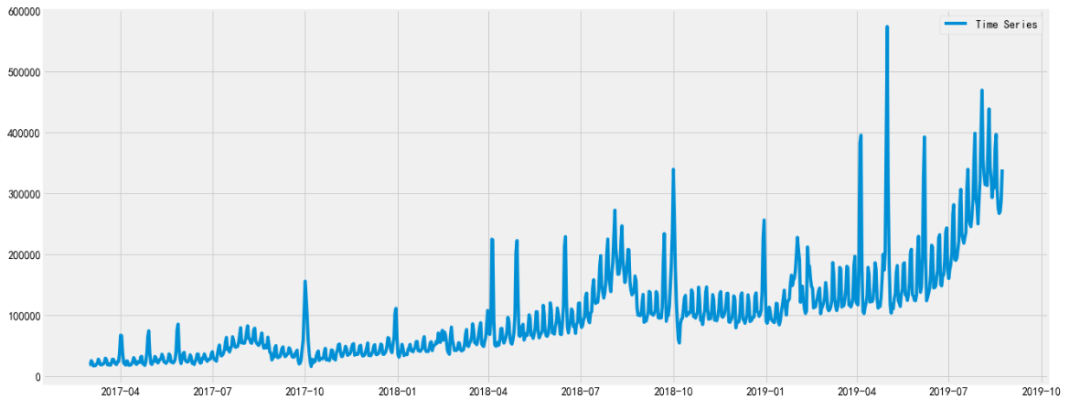Step2、时间序列平稳性检验

ARIMA 包含了 AR 模型，AR 模型的实质是用历史时间点数据预测当前时间点对应的值。这就要求序列的相关性不会随着时间变化而变化。

from statsmodels.tsa.stattools import adfullerdef test_stationarity(timeseries):    dftest = adfuller(timeseries, autolag='AIC')    return dftest

Step3、差分处理后检验平稳性

pred_day = 7train_start = datetime(2017,3,1)train_end = datetime(2019,8,16)pred_start = train_end+timedelta(1)pred_end = train_end+timedelta(pred_day) train_diff=df[train_start:train_end]train_diff['cnt']=train_diff.diff()print(test_stationarity(train_diff['cnt'][train_start+timedelta(1):train_end]))plt.figure(facecolor='white',figsize=(20,8))plt.plot(train_diff.index,train_diff['cnt'],label='Time Series after diff')plt.legend(loc='best')plt.show()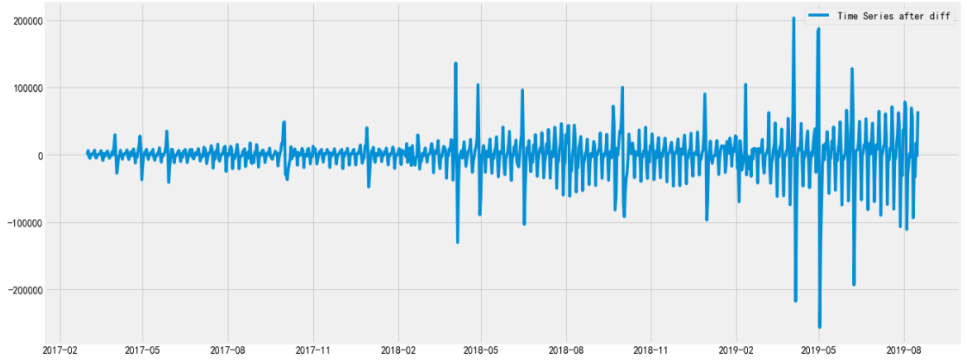Step4、画出 ACF 和 PACF 图

import statsmodels.api as smfig = plt.figure(figsize=(12,8))ax1 = fig.add_subplot(211)fig = sm.graphics.tsa.plot_acf(train_diff['cnt'][1:], lags=20, ax=ax1)ax1.xaxis.set_ticks_position('bottom')fig.tight_layout()ax2 = fig.add_subplot(212)fig = sm.graphics.tsa.plot_pacf(train_diff['cnt'][1:], lags=20, ax=ax2)ax2.xaxis.set_ticks_position('bottom')fig.tight_layout()plt.show()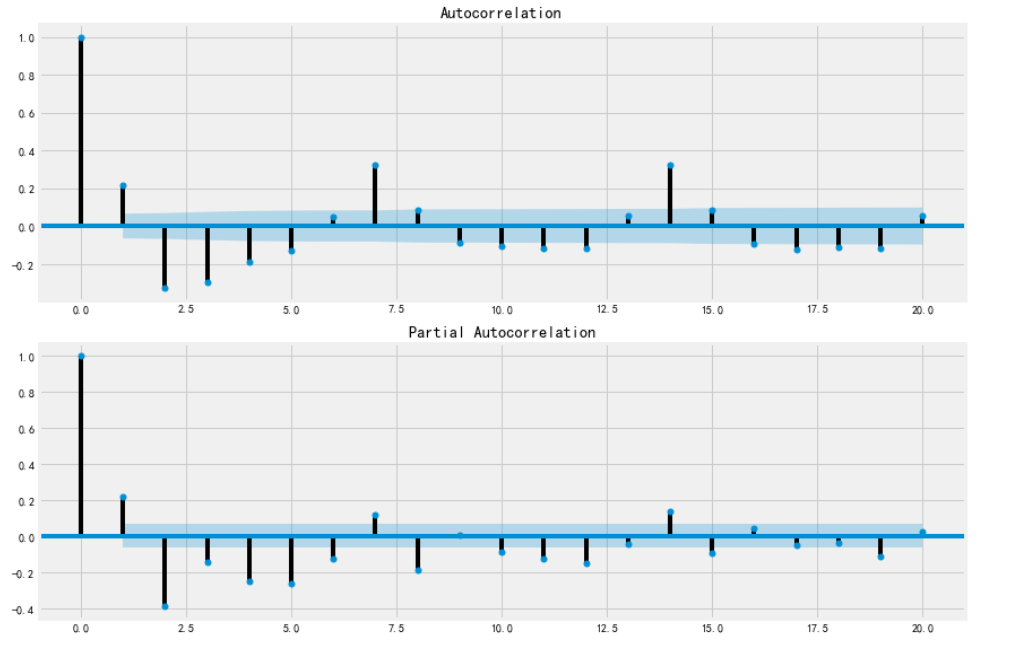Step5、ARIMA 模型定阶

#定阶warnings.filterwarnings("ignore") # specify to ignore warning messages pmax = 8qmax = 8aic_matrix = [] #aic 矩阵for p in range(1,pmax+1):    tmp = []    for q in range(1,qmax+1):        try: #存在部分报错，所以用 try 来跳过报错。            model = ARIMA(endog=df['cnt'],order=(p,1,q))            results = model.fit(disp=-1)            tmp.append(results.aic)            print('ARIMA p:{} q:{} - AIC:{}'.format(p, q, results.aic))        except:            tmp.append(None)    aic_matrix.append(tmp) aic_matrix = pd.DataFrame(aic_matrix) #从中可以找出最小值 p,q = aic_matrix.stack().idxmin() #先用 stack 展平，然后用 idxmin 找出最小值位置。print(u'AIC 最小的 p 值和 q 值为：%s、%s' %(p+1,q+1))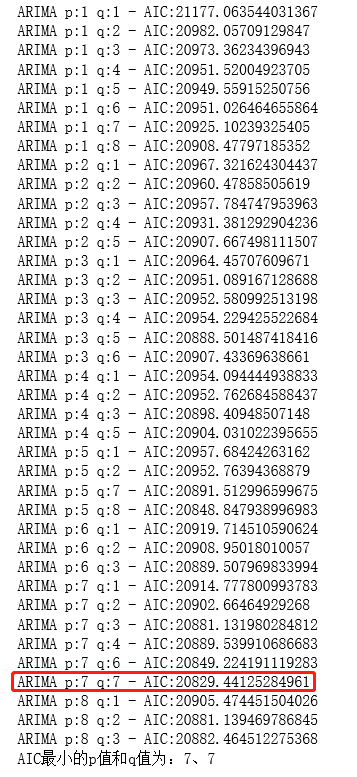Step6、模型测试与优化

model = ARIMA(endog=df['cnt'], order=(p,1,q))   #建立 ARIMA(7, 1,7) 模型result_ARIMA = model.fit(disp=-1,method='css') predict_diff=result_ARIMA.predict()#一阶差分还原df_shift=df['cnt'].shift(1)predict=predict_diff+df_shift plt.figure(figsize=(18,5),facecolor='white')predict[train_start+timedelta(p+1):train_end].plot(color='blue', label='Predict')df['cnt'][train_start+timedelta(p+1):train_end].plot(color='red', label='Original')err=sum(np.sqrt((predict[train_start+timedelta(p+1):train_end]-df['cnt'][train_start+timedelta(p+1):train_end])**2)/df['cnt'][train_start+timedelta(p+1):train_end])/df['cnt'][train_start+timedelta(p+1):train_end].sizeplt.legend(loc='best')plt.title('Error: %.4f'%err) plt.show()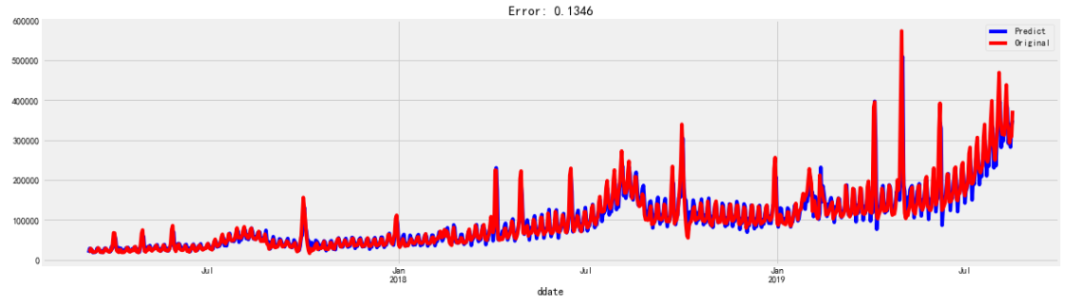y_forecasted =result_ARIMA.forecast(steps=pred_day, alpha=0.01) #作为期 7 天的预测y_truth = df[pred_start:pred_end]['cnt']# 均方根误差  #平均错误率mse = np.sqrt( ((y_forecasted - y_truth) ** 2) ).mean()error_rate = ( abs(y_forecasted - y_truth)/y_truth  ).mean()print('\nThe Mean Error rate of our forecasts is {}'.format(round(error_rate, 4)))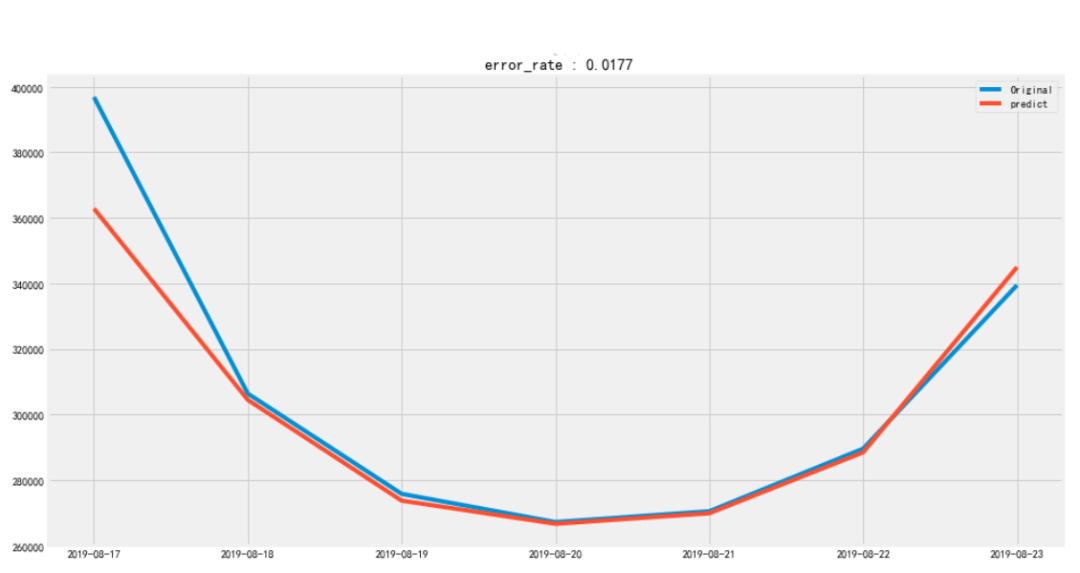Step7、模型检验

resid = result_ARIMA_improve.resid #赋值plt.figure(figsize=(12,8))qqplot(resid,line='q',fit=True)#利用 D-W 检验, 检验残差的自相关性print('D-W 检验值为{}'.format(durbin_watson(resid.values)))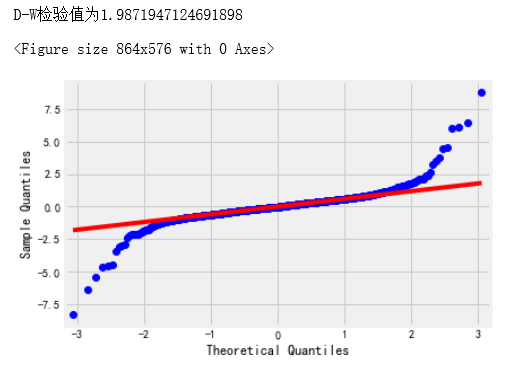## 三、总结与展望

• 对于时间序列的分析一定做好前期评估工作，直观的图表分析会助力我们的决策。多探索优秀的开源工具库，往往会使我们事半功倍。
• 模型选择至关重要，明确模型的适用场景，根据自身的时序选择适合的模型分析。
• ARIMA 模型在短时间内的预期效果还算可以，但是长时间比如未来一年的预测不太适用，因为偏差会逐渐增大。
• 现实中的复杂场景，单一模型很难解决，需要考虑多模型结合的方式实现分析预测。

June，携程数据分析经理，对数仓搭建，数据治理，数据分析等方面有较浓厚的兴趣。

2020 年 10 月 04 日 10:00 1668

## 评论

• ###### 时序分析在生物医学上的应用

几年前作者在学院的环境流行病课程上做了关于时序分析的演讲课程。这个课程的主要基调是：你应该忽略从时序模型、论文或者课本上读到的信息，说这个观点的原因是作者发现一些时序研究的相关文献在做生物医学和人口卫生环境分析方面并不是那么靠谱。

• ###### 蚂蚁金服 DASFAA 论文带你深入了解 GBDT 模型

GBDT应用的算法业务中对模型结果解释的需求日益增加。本文设计并解释了一种GBDT模型行之有效的局部解释性方案。

• ###### 41 丨数据挖掘实战（3）：如何对比特币走势进行预测？

今天我带你用数据挖掘对比特币的走势进行预测和分析，采用哪种方法比较好呢？

2019 年 3 月 18 日

• ###### Transformer 在美团搜索排序中的实践

本文介绍Transformer在美团搜索排序上的实践经验。

• ###### AI 工程师基础能力：机器学习

今天，我来和你聊一聊如何学习和掌握机器学习基础知识，又如何通过核心的知识脉络快速掌握更多的机器学习算法和模型。

AI
• ###### 无痛的增强学习入门：差分时序法

《无痛的增强学习入门》系列文章旨在为大家介绍增强学习相关的入门知识，为大家后续的深入学习打下基础。其中包括增强学习的基本思想，MDP框架，几种基本的学习算法介绍，以及一些简单的实际案例。 作为机器学习中十分重要的一支，增强学习在这些年取得了十分令人惊喜的成绩，这也使得越来越多的人加入到学习增强学习的队伍当中。增强学习的知识和内容与经典监督学习、非监督学习相比并不容易，而且可解释的小例子比较少，本系列将向各位读者简单介绍其中的基本知识，并以一个小例子贯穿其中。

• ###### 吴恩达团队提出倒计时回归模型：用 AI 技术预测病患死亡时间

近日，斯坦福大学Andrew Ng团队开发出了一种使用人工智能来预测病人死亡时间的系统。科学家希望该系统可以为病人提供更好的临床关怀。作者提出了一种新的预报检验方法Survival-CRPS，通过优化连续分级概率评分来提高预报的锐度，同时保持预报的校准度。这是科学界首次将最大似然法之外的评分方法成功应用于大型生存预测任务。

本文来自华为云开发者社区

• ###### 建模非正态分布：广义线性模型

今天我来和你分享广义线性模型的概念与原理，它克服了狭义线性模型的一些限制，拓展了线性模型的应用范围。

2018 年 7 月 10 日

• ###### 基于 LSTM 模型的广告库存预估算法

爱奇艺广告算法团队结合广告业务特点，提出基于LSTM（Long short-term memory，长短期记忆）模型的广告库存预估深度学习算法。

• ###### 周期性时间序列的预测

OpsDev，转载已获取作者授权。最近在研究时间序列的时候，发现很多序列具有很强的周期性，那如何对此类序列进行预测呢？

• ###### 频率视角下的机器学习

频率主义解决统计问题的基本思路如下：参数是确定的，数据是随机的，利用随机的数据推断确定的参数，得到的结果也是随机的。

2018 年 6 月 5 日

• ###### 那些在 Netflix Prize 中大放异彩的推荐算法

评分预测问题之所以虽然小众却十分重要，这一点得益于十多年前 Netflix Prize 的那一百万美元的悬赏效应。

2018 年 3 月 26 日

• ###### 导读：如何在机器学习中运用线性代数工具？

通过一个简单机器学习算法，讲解机器学习背后的线性代数真相，让你对线性代数有一个感性的认知。

2020 年 7 月 27 日

• ###### 携程金融自动化迭代反欺诈模型体系

本文介绍携程金融风控团队的自动化迭代反欺诈模型体系。

• ###### 统计意义（下）：如何通过显著性检验，判断你的 A/B 测试结果是不是巧合？

方差分析可以帮助我们检测差异的显著性，它分析的内容是受一个或多个因素影响的因变量在不同水平分组的差异。

2019 年 2 月 25 日

• ###### 如何评估 AI 在医学影像识别中的应用效果？

医学影像识别中常用的AI指标

kafka

Java

#### 做好仓储控制系统（WCS）的关键